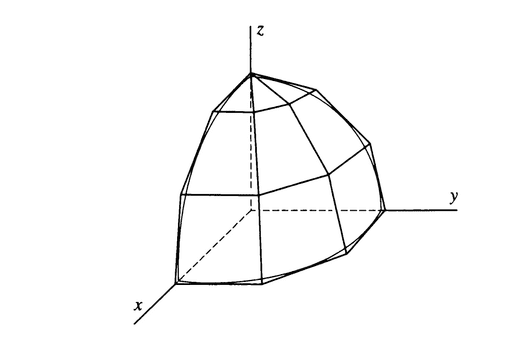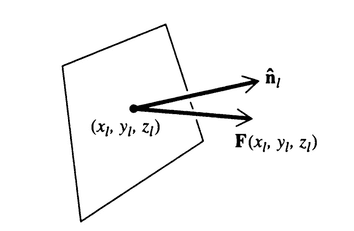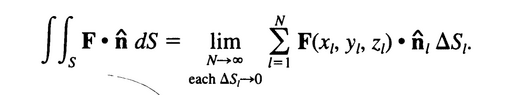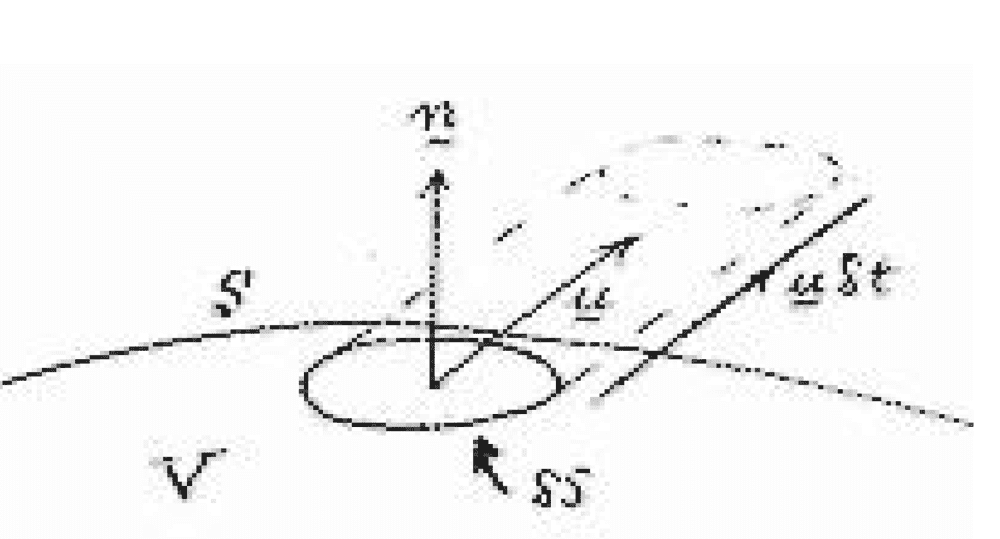# Doubt on the derivation of an equation for a surface integral

• B
• Hamiltonian
The dot product is used to find the component of the flux in a direction perpendicular to the surface. Multiplying by the surface area gives us the total flux.In summary, the method of derivation described in the conversation approximates a function using a polyhedron and focuses on one of its surfaces. The area of this surface is given by the dot product of the function and the unit normal vector, multiplied by the surface area. By adding all of these surfaces, the total flux of the vector field through the surface can be calculated. This concept is motivated by the idea of volumetric flow rates and is used to find the flux of a vector field through a surface, rather than finding the surface area itself.

#### Hamiltonian

TL;DR Summary
doubt in the derivation of the expression for calculating the surface integral for a function F.
this method of derivation is approximating the function using a polyhedron.concentrating on one of the surfaces(say the L'th surface which has an area ##\Delta S_l## and let ##(x_l,y_l,z_l)## be the coordinate of the point at which the face is tangent to the surface and let ##\hat n## be the unit normal vector)the area of this surface is given by ##F(x_l, y_l, z_l).\hat n_l \Delta S_l##
on adding all of these we get,my doubt in this derivation is why is there a dot product being taken between the vectors and then multiplied by the area(basically I don't understand why this equation, ##F(x_l, y_l, z_l).\hat n_l \Delta S_l## give the area as shouldn't the summation of all the ##\Delta S_l## simply be equal to the surface area of the function??)

Hamiltonian299792458 said:
the area of this surface is given by ##F(x_l, y_l, z_l).\hat n_l \Delta S_l##
on adding all of these we get,

No - the area of that little piece is just ##\Delta S_l##, whilst the (so-called) vector area of that little piece is ##\hat{n} \Delta S_l ##. The term ##\vec{F} \cdot \hat{n} \Delta S_l## is the "flux" of the vector field through that little piece, and by extension$$\Phi = \int_{\Sigma} \vec{F} \cdot \hat{n} dS$$is the flux out of the surface ##\Sigma##. For example, consider some fluid with velocity field ##\vec{v}(\vec{r})##. The volume of fluid that passes through the little piece of area ##dS## per time ##dt## is ##\vec{v} \cdot \hat{n} dS = (v\cos{\theta}) dS##. Note that this is the volume of an oblique prism of base area ##dS##, slant length ##v## and height ##v\cos{\theta}## between the faces at either end, which contains the fluid that has flown through the element per ##dt##.

The area of a surface is also ##\int_S dS##, whilst the vector area of a surface is ##\int_S \hat{n} dS##. N.B. that the vector area of a closed surface is zero.

[P.S. In many general cases, for a current density ##\vec{J} = \rho \vec{v}## associated with an extensive quantity ##Q## where ##\rho = \frac{\partial Q}{\partial V}##, if ´##Q## isn't being 'generated' or 'destroyed' inside the surface, you can say$$\frac{dQ_{\Omega}}{dt} = -\int_{\partial \Omega} \rho \vec{v} \cdot \hat{n} dS$$

•Hamiltonian
etotheipi said:
No - the area of that little piece is just ##\Delta S_l##, whilst the (so-called) vector area of that little piece is ##\hat{n} \Delta S_l ##. The term ##\vec{F} \cdot \hat{n} \Delta S_l## is the "flux" of the vector field through that little piece, and by extension$$\Phi = \int_{\Sigma} \vec{F} \cdot \hat{n} dS$$is the flux out of the surface ##\Sigma##.
I still don't understand howthis is derived.
(I understand the mistake in my previous post. how exactly do you get the surface area from the flux?)

Nothing's really been derived here, I think that's sort of the definition of the flux of a vector field through a surface. But I tried to give some motivation by looking at volumetric flow rates, maybe have a look at this figure:You'll notice that the volume inside that oblique cylinder is ##\vec{u} \delta t \cdot \hat{n} \delta S##, from which you might deduce the volumetric flow rate through a surface is ##\dot{V} = \int_{\Sigma} \vec{u} \cdot \hat{n} dS##.

Surface area is a simpler concept than flux, since the surface area of a surface is just ##\int_{\Sigma} dS##. If you want the vector surface area instead, then you can just consider ##\int_{\Sigma} \hat{n} dS##.

Last edited by a moderator:
•LCSphysicist and Hamiltonian
Hamiltonian299792458 said:
(I understand the mistake in my previous post. how exactly do you get the surface area from the flux?)

The main purpose of a surface integral is not to find a surface area. A surface integral computes the flux of a vector field through a surface.

Imagine we have pipe with a uniform cross section and water is flowing through it at a constant velocity. The flux of the water is measured in units like (liters per second)/ (square cm) and we would (theoretically) measure the flux by measuring the rate at which water flows through a surface that is a cross section of the pipe - i.e. a surface perpendicular to the sides of the pipe. Suppose we cannot measure the flow through a surface perpendicular to the sides of the pipe, but instead must measure it through a planar surface that is not perpedicular to the sides of the pipe. The dot product in a surface integral is used to correct for the fact we are not measuring the flow through a surface perpendicular to the sides of the pipe - or, as another way of looking at it, that we are not measuring flow in a direction perpendicular to the surface.

•Hamiltonian